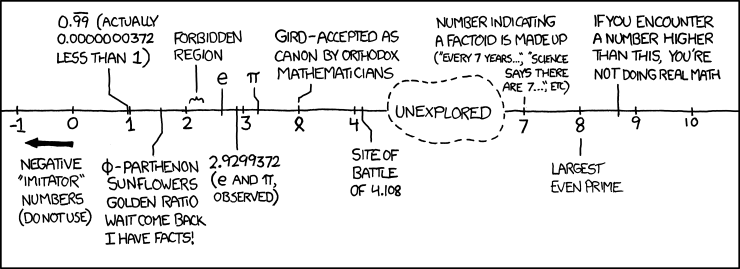Posted in Science & Nature

# Interesting Numbers

One of the more humorous sides to numbers is mathematicians’ attempts to categorise numbers as “interesting” or “dull”. For example, 1 is interesting because it is the first positive integer. 73 is interesting because it is the 21st prime number and 21 is a multiple of 7 and 3. The number 1729 is a good example of how a number can seem dull but later found to be interesting. When the British mathematician G. H. Hardy visited Indian mathematician Srinivasa Ramanujan, he commented that the number of the taxicab he rode in on was 1729 – a number he found to be rather dull. Ramanujan objected and stated it is very interesting as it is the smallest number expressible as the sum of two cubes in two different ways (1729 = 1³ + 12³ = 9³ + 10³). Such numbers are now referred to as taxicab numbers and 1729 is called the Hardy-Ramanujan number.

A way to discover the smallest most uninteresting number is through the Online Encyclopaedia of Integer Sequences, which documents every integer worth noting as it is in some sort of arithmetic sequence. The smallest integer that does not appear in this encyclopaedia as part of a sequence could be considered as objectively the smallest “uninteresting” number. In 2009, this number was 11630, but has since changed to 12407, then 13794 and now 14228 (22 April 2014).

But paradoxically, the smallest uninteresting number is interesting in itself by being the smallest most uninteresting number. This is known as the interesting number paradox. By this paradox, every natural number is unique and ergo, “interesting”.(Image source: http://www.xkcd.com/899/, and here’s an explanation of some of the numbers on it)